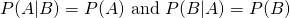## Probability

 Addition Rule for Disjoint Events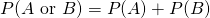General Addition Rule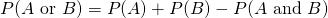Complement If set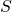= {1,2,3} and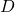= {1} Then the complement is:= {2,3} Multiplication Rule for Independent Events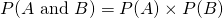Conditional Probability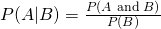General Multiplication Rule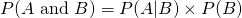Bayes' Theorem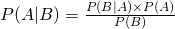Independence RuleIndependence Rule